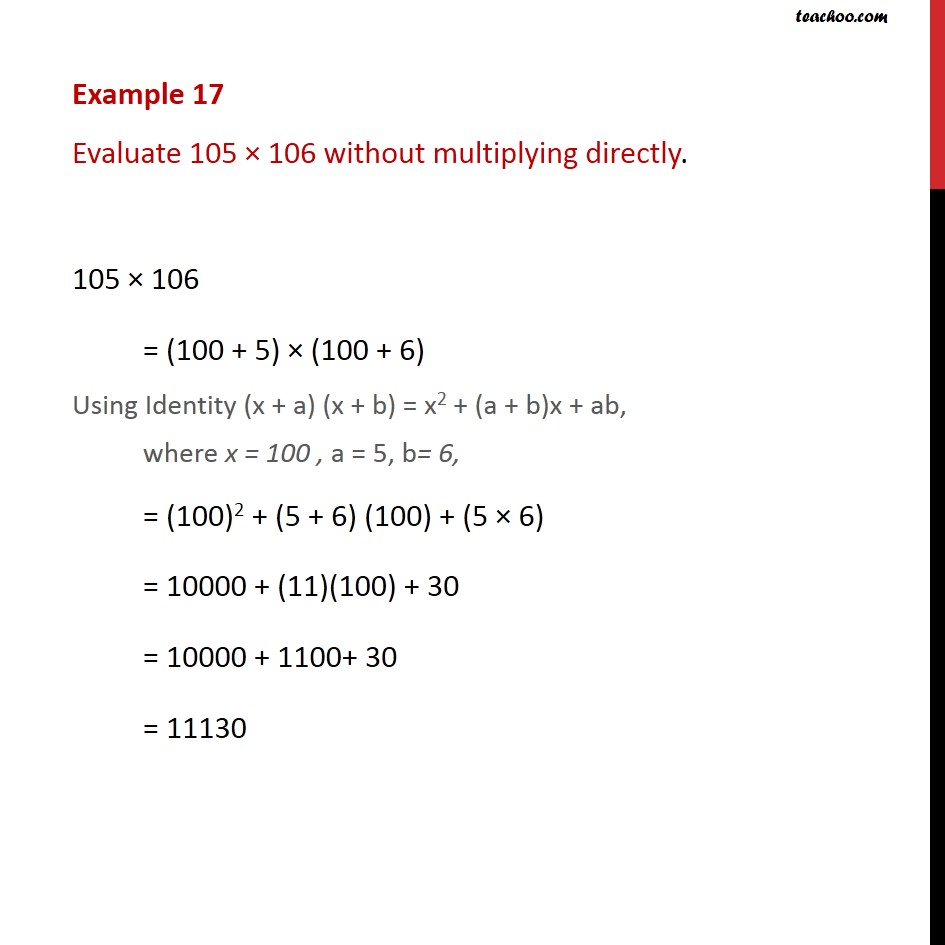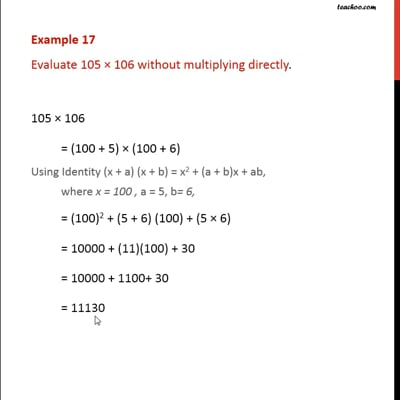Examples

Chapter 2 Class 9 Polynomials
Serial order wiseThis video is only available for Teachoo black users

Get live Maths 1-on-1 Classs - Class 6 to 12

### Transcript

Example 17 Evaluate 105 × 106 without multiplying directly. 105 × 106 = (100 + 5) × (100 + 6) Using Identity (x + a) (x + b) = x2 + (a + b)x + ab, where x = 100 , a = 5, b= 6, = (100)2 + (5 + 6) (100) + (5 × 6) = 10000 + (11)(100) + 30 = 10000 + 1100+ 30 = 11130